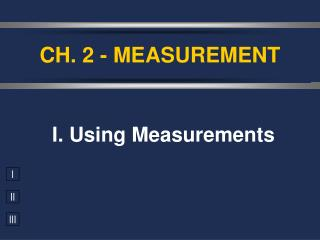DownloadDownload PresentationCH. 2 - MEASUREMENT

# CH. 2 - MEASUREMENT

Download Presentation## CH. 2 - MEASUREMENT

- - - - - - - - - - - - - - - - - - - - - - - - - - - E N D - - - - - - - - - - - - - - - - - - - - - - - - - - -
##### Presentation Transcript

1. CH. 2 - MEASUREMENT I. Using Measurements

2. A. Accuracy vs. Precision • Accuracy - how close a measurement is to the accepted value • Precision - how close a series of measurements are to each other ACCURATE = CORRECT PRECISE = CONSISTENT

3. Accurate but not precise. The average is close to the center but the individual values are not similar

4. Precise, but not accurate All measurements are close, but not at the “correct” value

5. Accurate AND Precise All measurements are close, AND at the “correct” value

6. Identify each

7. your value accepted value B. Percent Error • Indicates accuracy of a measurement

8. % error = 2.9 % B. Percent Error • A student determines the density of a substance to be 1.40 g/mL. Find the % error if the accepted value of the density is 1.36 g/mL.

9. C. Significant Figures • Indicate precision of a measurement. • Recording Sig Figs • Sig figs in a measurement include the known digits plus a final estimated digit 2.35 cm

10. C. Significant Figures • Counting Sig Figs(p. 4 of Unit 1 packet) • Count all numbers EXCEPT: • Leading zeros -- 0.0025 • Trailing zeros without a decimal point -- 2,500

11. Atlantic Pacific Rule • If the decimal is ABSENT, start from the ATLANTIC side, go to the first non-zero digit and start counting. • If the decimal is PRESENT, start from the PACIFIC side, go to the first non-zero digit and start counting. Atlantic Pacific

12. C. Significant Figures Counting Sig Fig Examples 1. 23.50 1. 23.50 4 sig figs 3 sig figs 2. 402 2. 402 3. 5,280 3. 5,280 3 sig figs 2 sig figs 4. 0.080 4. 0.080

13. C. Significant Figures • Calculating with Sig Figs • Add/Subtract - The # with the lowest decimal value determines the place of the last sig fig in the answer. 224 g + 130 g 354 g 3.75 mL + 4.1 mL 7.85 mL 3.75mL + 4.1mL 7.85 mL  7.9 mL  354 g

14. 3 SF C. Significant Figures • Calculating with Sig Figs (con’t) • Multiply/Divide - The # with the fewest sig figs determines the # of sig figs in the answer. (13.91g/cm3)(23.3cm3) = 324.103g 4 SF 3 SF 324g

15. C. Significant Figures • Calculating with Sig Figs (con’t) • Exact Numbers do not limit the # of sig figs in the answer. • Counting numbers: 12 students • Exact conversions: 1 m = 100 cm • “1” in any conversion: 1 in = 2.54 cm

16. 5. (15.30 g) ÷ (6.4 mL)  2.4 g/mL 2 SF C. Significant Figures Practice Problems 4 SF 2 SF = 2.390625 g/mL 6. 18.9 g - 0.84 g  18.1 g 18.06 g

17. D. Scientific Notation • Converting into Sci. Notation: • Move decimal until there’s 1 digit to its left. Places moved = exponent. • Large # (>1)  positive exponentSmall # (<1)  negative exponent • Only include sig figs. 65,000 kg  6.5 × 104 kg

18. 7. 2,400,000 g 8. 0.00256 kg 9. 7  10-5 km 10. 6.2  104 mm D. Scientific Notation Practice Problems 2.4  106 g 2.56  10-3 kg 0.00007 km 62,000 mm

19. EXE EXP EXP ENTER EE EE D. Scientific Notation • Calculating with Sci. Notation (5.44 × 107 g) ÷ (8.1 × 104 mol) = Type on your calculator: 5.44 7 8.1 4 ÷ = 671.6049383 = 670 g/mol = 6.7 × 102 g/mol